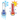# Specific Heat Calculator

## Specific Heat

Energy

J

Mass

Kg

Temperatureinitial

°C

Temperaturefinal

°C

Specific Heat

J/Kg.C### Specific Heat

Heat is the amount of energy streaming from one body of matter to another automatically due to their temperature variation. The specific heat capacity (specific heat), is the heat capacity per unit mass of material.

### Specific Heat Formula

Q = total energy,m = mass, c = heat.
c = Q / m x change in Temperature
Q = c x m x change in Temperature

First, discover the total amount of energy being placed into the system. measure the mass of the object you are testing/calculating, measure the variation in temperature of the object. When you get into ambient lost heat, these equations of systems get much more confused, but for a simple experiment just measure the temperature of the object is enough, finally calculated.